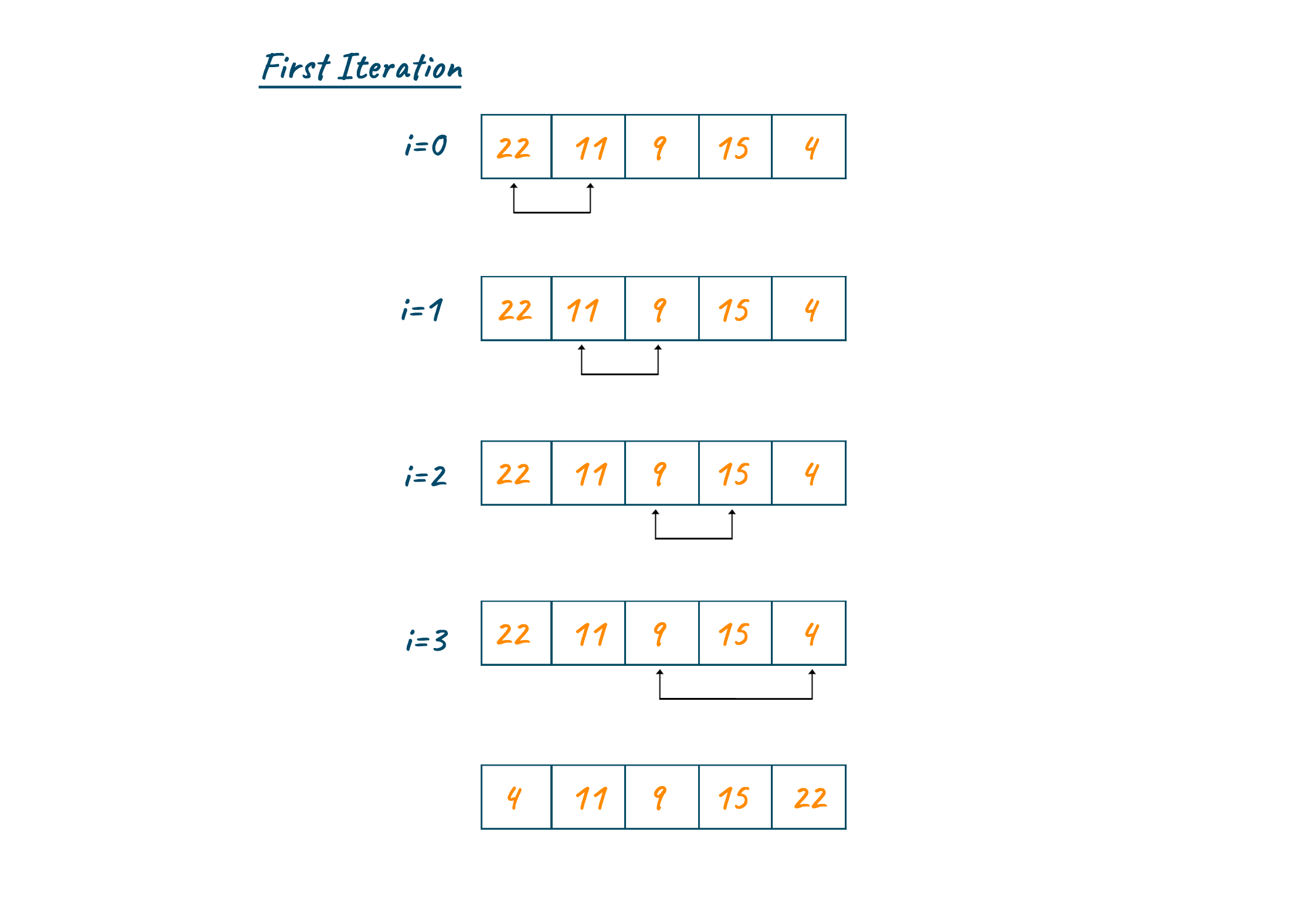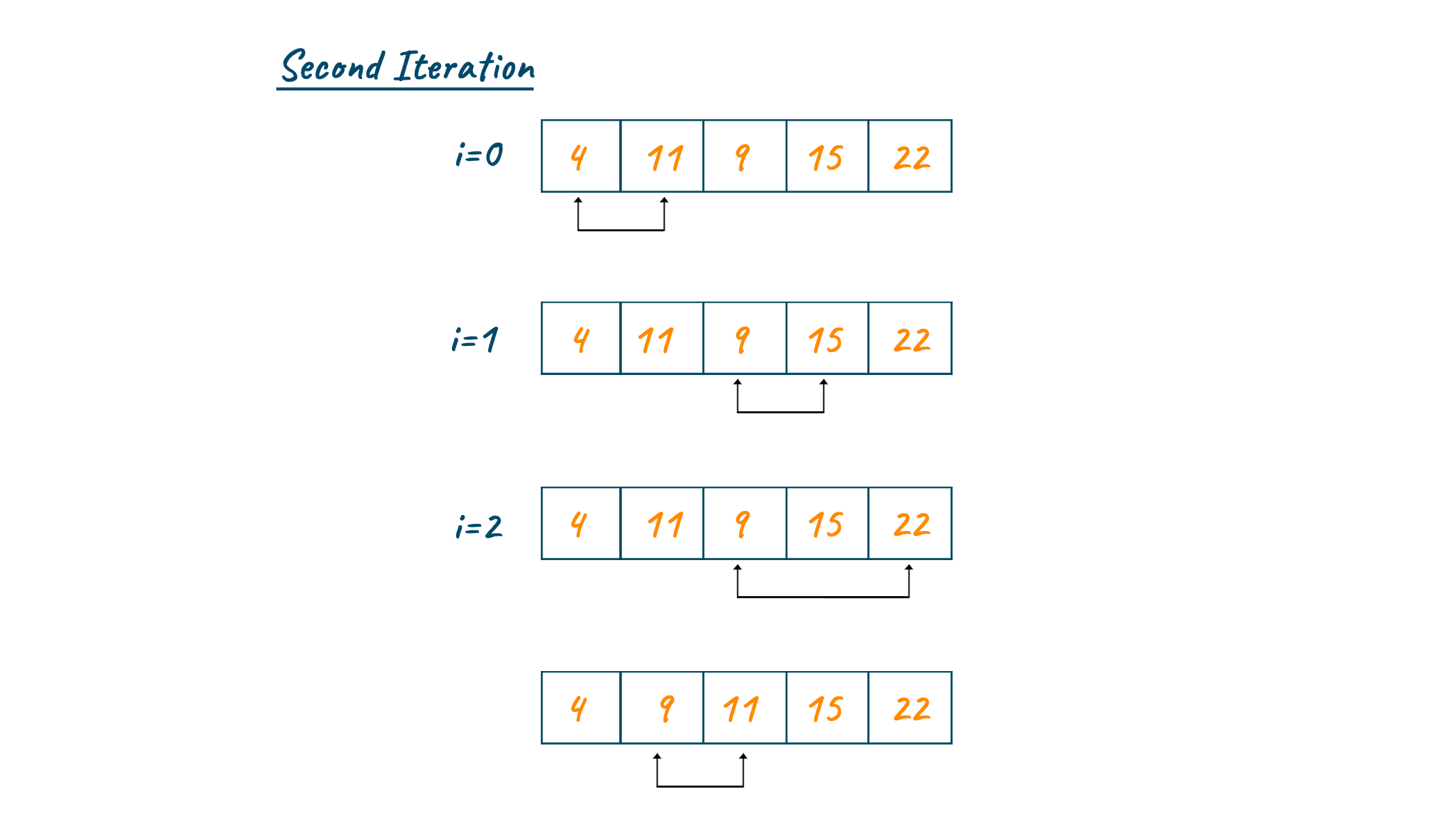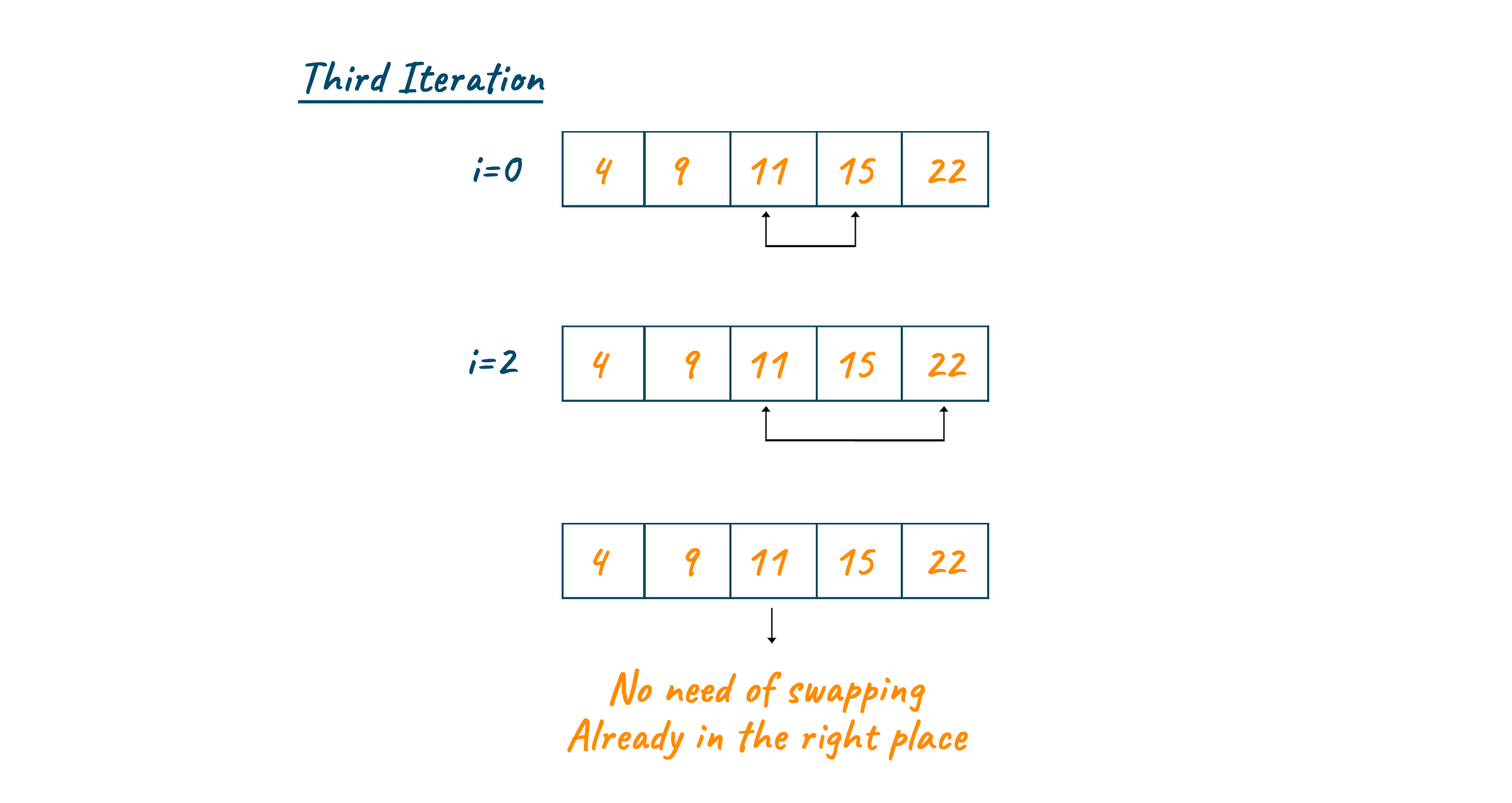# Selection Sort in C++: Example & Advantages (with code)

Sorting algorithms take input data in the form of lists of items, and produce an ordered list. One of the simplest ones is Selection Sort in C++, which we will study in this article. We will also provide you with a C++ program to implement Selection Sort.

## What is Selection Sort in C++?

Selection sort is a sorting algorithm that selects the smallest element from an unsorted list and sets it at the beginning of the unsorted list in each iteration. It sorts an array by repeatedly choosing the smallest member from the unsorted segment and placing it at the beginning (in ascending order).

Selection sort uses in-place comparisons to sort data. The array or list is separated into two parts during the selection sort algorithm: the sorted part on the left end and the unsorted part on the right end. The sorted component is initially empty, while the unsorted part is the complete array or list.

### Pseudocode for Selection Sort

```selectionSort(array, size)
repeat (size - 1) times
set the first unsorted element as the minimum
for each of the unsorted elements
if element < currentMinimum
set element as new minimum
swap minimum with first unsorted position
end selectionSort
```

### How does it Work?

Let’s understand the working of selection sort step by step with an example:

• Step 1: Set the array's first element as the minimum.
• Step 2: Compare the first and second elements. Assign the second element as a minimum if it is smaller than the first.
• Step 3: Compare the third element to the minimum. If the third element is smaller, assign it as a minimum; otherwise, don’t do any changes. The process continues until the final element of the array.
• Step 4: Minimum is moved to the front of the unsorted list after each iteration.
• Step 5: Indexing begins with the first unsorted element in each iteration. Steps are repeated until all the elements are placed at their correct positions.

Now, let's look at a simple example. Looking at the below image you can see that we will compare each element throughout the array and find the first minimum. Assign the first minimum to the first index of an array after the completion of the first pass.Repeating the same process, we will traverse the array and find the second minimum. Later assign the second minimum to the second index of an array as shown in the below image.Keep repeating the same process. In our example, the elements are already in the right position and therefore no need for swapping is required.### Is Selection Sort Algorithm stable?

If an algorithm maintains the relative order of the same entries in a sorted list, we can say it is stable. If two elements have the same value in the sequence, the algorithm should sort them according to another attribute.

Selection Sort Algorithm is not Stable. This swapping operation in the process may change the relative order of elements with equal values. It is not reliable as well due to the same fact. This is due to the fact that after sorting, it does not guarantee to preserve the relative order of identical elements in the sequence of inputs.

### Is Selection Sort the Fastest?

The quickest sorting algorithm in C++ is not a selection sort. It is slower than other sorting algorithms like quicksort and mergesort, with an O(n2) time complexity for both average and worst-case scenarios.

In C++, quicksort and mergesort are two alternative sorting algorithms that are quicker than selection sort. Mergesort has an O(n log n) worst-case time complexity, while Quicksort has an O(n log n) average-case time complexity.  Each of these algorithms benefits from partially ordered input sequences because they are adaptive and flexible.

## C++ Program for Selection Sort

Let’s understand the C++ program to implement selection sort. Here is the complete code:

```#include<bits/stdc++.h>
using namespace std;
void print(int arr[], int n)
{
for(int i=0;i<n;i++)
cout<<arr[i]<<" ";
cout<<endl;
}
void selectionSort(int arr[], int n)
{
int i,j,min_in;
for(i=0;i<n;i++)
{
min_in = i;
for(j=i+1;j<n;j++)
if (arr[j] < arr[min_in])
min_in = j;
swap(arr[i],arr[min_in]);
}
}
int main(int argv, char* argc[])
{
int arr[] = {5,4,10,1,6,2};
int i,j,n,temp;
n = sizeof(arr)/sizeof(int);
cout<<"Unsorted Array :";
print(arr,n);
selectionSort(arr,n);
cout<<"Sorted Array :";
print(arr,n);
return(0);
}
```

Output:

```Unsorted Array :5 4 10 1 6 2
Sorted Array :1 2 4 5 6 10
```

### Time Complexity

The Average Case Complexity of Selection Sort in C++ is O(n2). It happens when the array elements are in a jumbled sequence that isn't ascending or descending properly. Other than that, the bese case complexity and worst case complexity are O(n2) as well.

Compared with other sorting algorithms, selection sort is easier to use. It is great when to be used for shorter inputs. It is also space efficient since it operates directly on the input array. But a big drawback is its time complexity. It is inefficient for enormous input sizes because its worst-case complexity for time is O(n2).

Also, we discussed that it is not unstable and that t might alter the relative order of identical elements in the sequence. We also see its inefficiency if the input array is partially sorted, the algorithm will still perform the same number of comparisons. Bubble Sort is a better alternative in this scenario.

## Conclusion

In summary, we covered all about selection sort in C++ with a complete program to implement it. Remember that the principle of the selection sort is to find the smallest element in the array and place it in the correct position every time. Happy Learning :)

### FavTutor - 24x7 Live Coding Help from Expert Tutors!